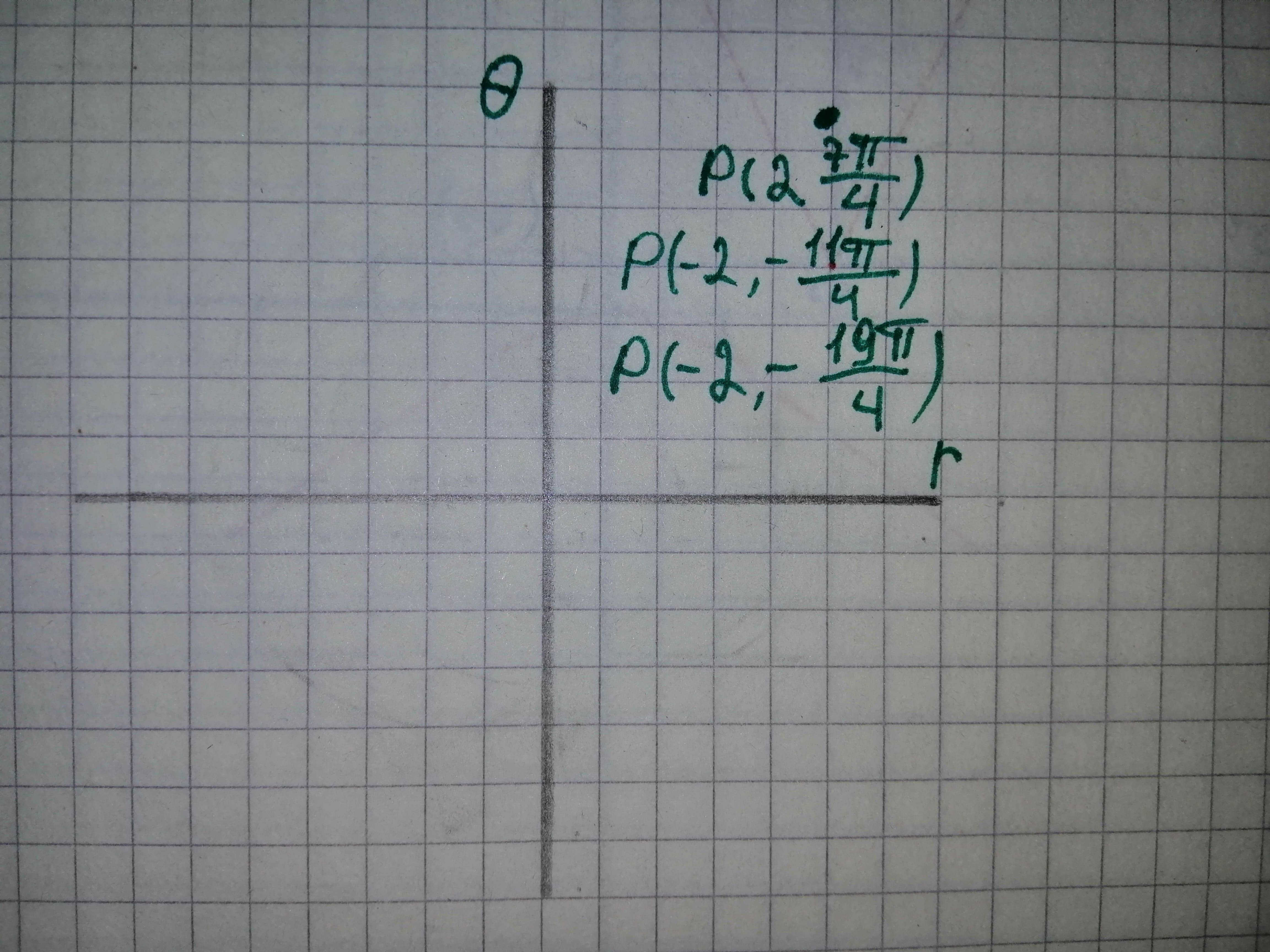# To plot: Thepoints which has polar coordinate displaystyle{left({2},frac{{{7}pi}}{{4}}right)} also two alternaitve sets for the same.Suman Cole 2021-03-02 Answered
To plot: Thepoints which has polar coordinate $\left(2,\frac{7\pi }{4}\right)$ also two alternaitve sets for the same.
You can still ask an expert for help

• Questions are typically answered in as fast as 30 minutes

Solve your problem for the price of one coffee

• Math expert for every subject
• Pay only if we can solve itDora

Let the point $\left(2,\frac{7\pi }{4}\right)$ be denoted by P.
Consider the point $\left(2,\frac{7\pi }{4}\right)$, it is 2 units from the Origin O and line OP makes an angle of $\frac{7\pi }{4}$ with the positive direction of the x-axis.
Add pi to the angle and change cardinal coordinate to -2 to get one alternate solution.
Therefore, the point P $\left(2,\frac{7\pi }{4}\right)$ can also be represented by $\left(-2,\frac{7\pi }{4}+\pi \right)=\left(-2,\frac{11\pi }{4}\right).$
Change the radial coordinate to -2 and subtract $3\pi$ from the angle to obtain the other alternate solution.
Thus, the point $P\left(2,\frac{7\pi }{4}\right)$ can be given by $\left(-2,\frac{7\pi }{4}-3\pi \right)$
$=\left(-2,-\frac{19\pi }{4}\right).$
Use online graphing calculator and plot the points of P as shown below in Figure 1.From the Figure 1 it can be noted that alternate representations are plotted on the same location.